# 【知识蒸馏】Deep Mutual Learning¶

【GiantPandaCV导语】Deep Mutual Learning是Knowledge Distillation的外延，经过测试（代码来自Knowledge-Distillation-Zoo), Deep Mutual Learning性能确实超出了原始KD很多，所以本文分析这篇CVPR2018年被接受的论文。同时PPOCRv2中也提到了DML，并提出了CML，取得效果显著。

## 引言¶

• logits(mimic learning via regressing logits): + 0.78

• ST(soft target): + 0.16

• OFD(overhaul of feature distillation): +0.45

• AT(attention transfer): +0.71

• NST(neural selective transfer): +0.38

• RKD(relational knowledge distillation): +0.65

• AFD(attention feature distillation): +0.18

• DML(deep mutual learning): + 2.24 （ps: 这里教师网络已经训练好了，与DML不同）

DML也是传统知识蒸馏的扩展，其目标也是将大型模型压缩为小的模型。但是不同于传统知识蒸馏的单向蒸馏（教师→学生），DML认为可以让学生互相学习（双向蒸馏），在整个训练的过程中互相学习，通过这种方式可以提升模型的性能。

DML通过实验证明在没有先验强大的教师网络的情况下，仅通过学生网络之间的互相学习也可以超过传统的KD。

## DML¶

Deep Mutual Learning则是让两个小的学生网络同时学习，对于每个单独的网络来说，会有针对hard label的分类损失函数，还有模仿另外的学生网络的损失函数，用于对齐学生网络的类别后验。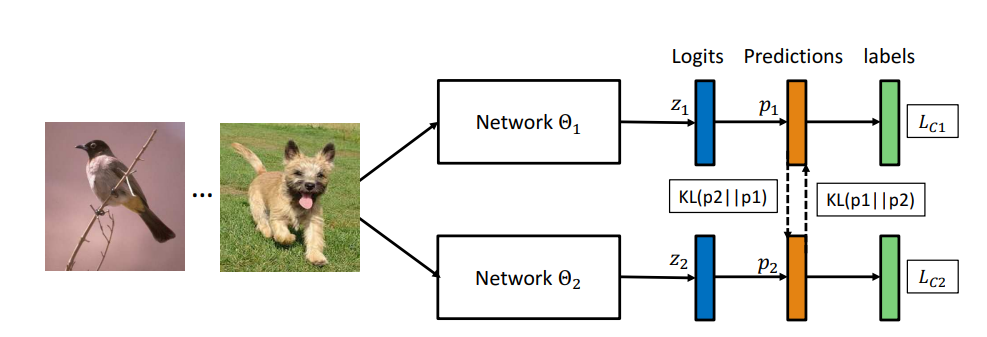• 每个学生主要是倍传统的有监督学习损失函数影响，这意味着学生网络的性能大体会是增长趋势，这意味着他们的表现通常会提高，他们不能作为一个群体任意地漂移到群体思维。（原文： they cannot drift arbitrarily into groupthink as a cohort.）

• 在监督信号下，所有的网络都会朝着预测正确label的方向发展，但是不同的网络在初始化值不同，他们会学到不同的表征，因此他们对下一类最有可能的概率的估计是不同的。

• 在Mutual Learning中，学生群体可以有效汇集下一个最后可能的类别估计，为每个训练实例找到最有可能的类别，同时根据他们互学习对象增加每个学生的后验熵，有助于网络收敛到更平坦的极小值，从而带来更好的泛华能力和鲁棒性。

• Why Deep Nets Generalise 有关网络泛化性能的讨论认为：在深度神经网络中，有很多解法（参数组合）可以使得训练错误为0，其中一些在比较loss landscape平坦处参数可以比其他narrow位置的泛华性能更好，所以小的干扰不会彻底改变预测的效果;

• DML通过实验发现：（1）训练过程损失可以接近于0 。（2）在扰动下对loss的变动接受能力更强。（3）给出的class置信度不会过于高。总体来说就是：DML并没有帮助我们找到更好的训练损失最小值，而是帮助我们找到更广泛/更稳健的最小值，更好地对测试数据进行泛华。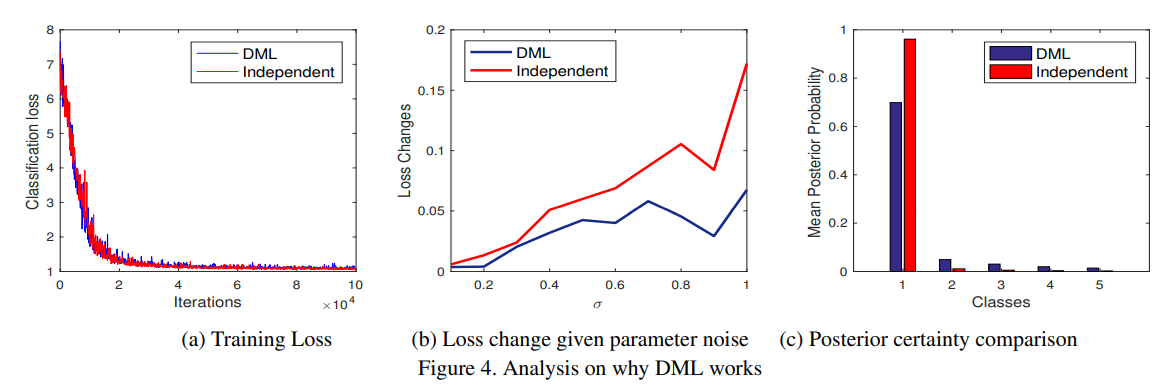DML具有的特点是：

• 适合于各种网络架构，由大小网络混合组成的异构的网络也可以进行相互学习（因为只学习logits）

• 效能会随着队列中网络数量的增加而增加，即互学习对象增多的时候，性能会有一定的提升。

• 有利于半监督学习，因为其在标记和未标记数据上都激活了模仿损失。

• 虽然DML的重点是得到某一个有效的网络，整个队列中的网络可以作为模型集成的对象进行集成。

DML中使用到了KL Divergence衡量两者之间的差距：

D_{K L}\left(\boldsymbol{p}_{2} \| \boldsymbol{p}_{1}\right)=\sum_{i=1}^{N} \sum_{m=1}^{M} p_{2}^{m}\left(\boldsymbol{x}_{i}\right) \log \frac{p_{2}^{m}\left(\boldsymbol{x}_{i}\right)}{p_{1}^{m}\left(\boldsymbol{x}_{i}\right)}

P1和P2代表两者的逻辑层输出，那么对于每个网络来说，他们需要学习的损失函数为：

\begin{aligned} &L_{\Theta_{1}}=L_{C_{1}}+D_{K L}\left(\boldsymbol{p}_{2} \| \boldsymbol{p}_{1}\right) \\ &L_{\Theta_{2}}=L_{C_{2}}+D_{K L}\left(\boldsymbol{p}_{1} \| \boldsymbol{p}_{2}\right) \end{aligned}

\frac{1}{2}\left(D_{K L}\left(\boldsymbol{p}_{1} \| \boldsymbol{p}_{2}\right)+D_{K L}\left(\boldsymbol{p}_{1} \| \boldsymbol{p}_{2}\right)\right)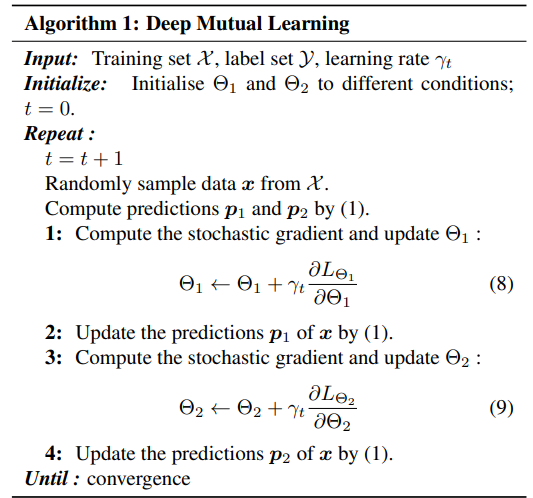L_{\Theta_{k}}=L_{C_{k}}+\frac{1}{K-1} \sum_{l=1, l \neq k}^{K} D_{K L}\left(\boldsymbol{p}_{l} \| \boldsymbol{p}_{k}\right)

## 实验结果¶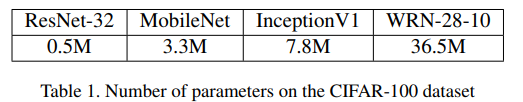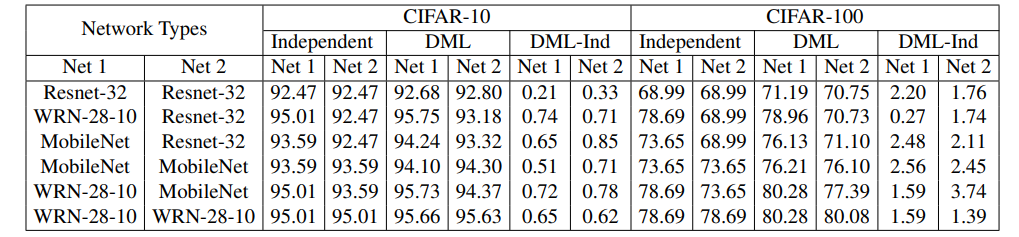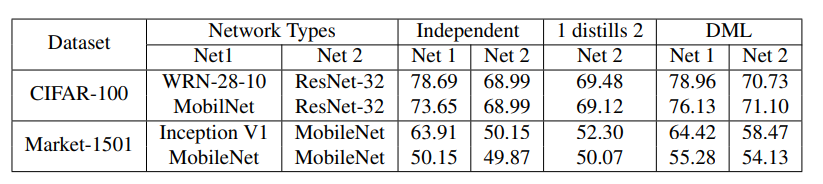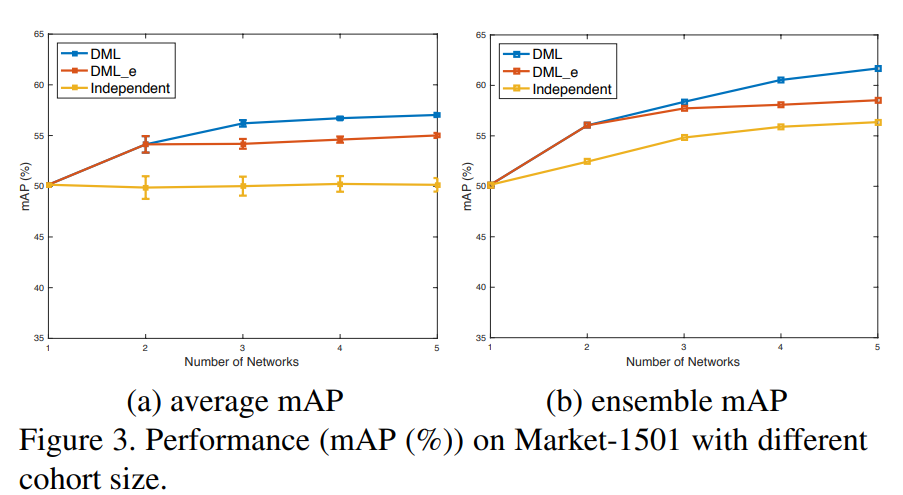## 参考¶

https://openaccess.thecvf.com/content_cvpr_2018/papers/Zhang_Deep_Mutual_Learning_CVPR_2018_paper.pdf